图像处理中阈值的设置_图像处理阈值化的阈值确定 - CSDN

•  Otsu（大津法或最大类间方差法）使用的是聚类的思想，把图像的灰度数按灰度级分成2个部分，使得两个部分之间的灰度值差异最大，每个部分之间的灰度差异最小，通过方差的计算来寻找一个合适的灰度级别来划分。...
目录

1、Otsu阈值分割

2、自适应阈值分割

3、 最大熵阈值分割法

4、 迭代阈值分割

5、测验

1、Otsu阈值分割

Otsu（大津法或最大类间方差法）使用的是聚类的思想，把图像的灰度数按灰度级分成2个部分，使得两个部分之间的灰度值差异最大，每个部分之间的灰度差异最小，通过方差的计算来寻找一个合适的灰度级别来划分。 所以可以在二值化的时候采用otsu算法来自动选取阈值进行二值化。otsu算法被认为是图像分割中阈值选取的最佳算法，计算简单，不受图像亮度和对比度的影响。因此，使类间方差最大的分割意味着错分概率最小。

参考链接：https://www.cnblogs.com/moon1992/p/5092726.html

opencv调用格式为：threshold(sourceImage, dstImage, 0, 255, CV_THRESH_OTSU);

sourceImage：输入图像；

dstImage：输出图像；

otsu算法的源码如下：

Mat OtsuAlgThreshold(Mat &image)
{
if (image.channels() != 1)
{
cout << "Please input Gray-image!" << endl;
}
int T = 0; //Otsu算法阈值
double varValue = 0; //类间方差中间值保存
double w0 = 0; //前景像素点数所占比例
double w1 = 0; //背景像素点数所占比例
double u0 = 0; //前景平均灰度
double u1 = 0; //背景平均灰度
double Histogram = { 0 }; //灰度直方图，下标是灰度值，保存内容是灰度值对应的像素点总数
uchar *data = image.data;

double totalNum = image.rows*image.cols; //像素总数

for (int i = 0; i < image.rows; i++)
{
for (int j = 0; j < image.cols; j++)
{
if (image.at<uchar>(i, j) != 0) Histogram[data[i*image.step + j]]++;
}
}
int minpos, maxpos;
for (int i = 0; i < 255; i++)
{
if (Histogram[i] != 0)
{
minpos = i;
break;
}
}
for (int i = 255; i > 0; i--)
{
if (Histogram[i] != 0)
{
maxpos = i;
break;
}
}

for (int i = minpos; i <= maxpos; i++)
{
//每次遍历之前初始化各变量
w1 = 0;       u1 = 0;       w0 = 0;       u0 = 0;
//***********背景各分量值计算**************************
for (int j = 0; j <= i; j++) //背景部分各值计算
{
w1 += Histogram[j];   //背景部分像素点总数
u1 += j*Histogram[j]; //背景部分像素总灰度和
}
if (w1 == 0) //背景部分像素点数为0时退出
{
break;
}
u1 = u1 / w1; //背景像素平均灰度
w1 = w1 / totalNum; // 背景部分像素点数所占比例
//***********背景各分量值计算**************************

//***********前景各分量值计算**************************
for (int k = i + 1; k < 255; k++)
{
w0 += Histogram[k];  //前景部分像素点总数
u0 += k*Histogram[k]; //前景部分像素总灰度和
}
if (w0 == 0) //前景部分像素点数为0时退出
{
break;
}
u0 = u0 / w0; //前景像素平均灰度
w0 = w0 / totalNum; // 前景部分像素点数所占比例
//***********前景各分量值计算**************************

//***********类间方差计算******************************
double varValueI = w0*w1*(u1 - u0)*(u1 - u0); //当前类间方差计算
if (varValue < varValueI)
{
varValue = varValueI;
T = i;
}
}
Mat dst;
threshold(image, dst, T, 255, CV_THRESH_OTSU);
return dst;
}

2、自适应阈值分割

二值化算法是用输入像素的值I与一个值C来比较，根据比较结果确定输出值。

自适应二值化的每一个像素的比较值C都不同，比较值C由这个像素为中心的一个块范围计算在减去差值delta得到。

其中，C的常用计算方法有两种：

a、平均值减去差值delta（使用盒过滤boxfilter，性能会非常不错）

b、高斯分布加权和减去差值delta (使用高斯滤波GaussionBlur)

void myadaptive(InputArray _src, OutputArray _dst, double maxValue,
int method, int type, int blockSize, double delta)
{
Mat src = _src.getMat();

CV_Assert(src.type() == CV_8UC1);
CV_Assert(blockSize % 2 == 1 && blockSize > 1);
Size size = src.size();

_dst.create(size, src.type());
Mat dst = _dst.getMat();

if (maxValue < 0)
{
dst = Scalar(0);
return;
}

Mat mean;
if (src.data != dst.data)
mean = dst;
{
GaussianBlur(src, mean, Size(blockSize, blockSize), 0, 0, BORDER_REPLICATE);
}
{
boxFilter(src, mean, src.type(), Size(blockSize, blockSize),
Point(-1, -1), true, BORDER_REPLICATE);
}
else
{
}

int i, j;
uchar imaxval = saturate_cast<uchar>(maxValue);
int idelta = type == THRESH_BINARY ? cvCeil(delta) : cvFloor(delta);
uchar tab;

if (type == CV_THRESH_BINARY)
for (i = 0; i < 768; i++)
tab[i] = (uchar)(i - 255 > -idelta ? imaxval : 0);
else if (type == CV_THRESH_BINARY_INV)
for (i = 0; i < 768; i++)
tab[i] = (uchar)(i - 255 <= -idelta ? imaxval : 0);
else
{
}

if (src.isContinuous() && mean.isContinuous() && dst.isContinuous())
{
size.width *= size.height;
size.height = 1;
}

for (i = 0; i < size.height; i++)
{
const uchar* sdata = src.data + src.step*i;
const uchar* mdata = mean.data + mean.step*i;
uchar* ddata = dst.data + dst.step*i;

for (j = 0; j < size.width; j++)
// 将[-255, 255] 映射到[0, 510]然后查表
ddata[j] = tab[sdata[j] - mdata[j] + 255];
}
}

3、 最大熵阈值分割法

参考链接：https://blog.csdn.net/qq_27668313/article/details/77949596

https://blog.csdn.net/robin__chou/article/details/53931442

https://blog.csdn.net/xw20084898/article/details/22760169

最大熵阈值分割函数源码如下：

Mat EntropySeg(Mat src)
{
int tbHist = { 0 };
int index = 0;
double Property = 0.0;
double maxEntropy = -1.0;
double frontEntropy = 0.0;
double backEntropy = 0.0;
int TotalPixel = 0;
int nCol = src.cols*src.channels();
for (int i = 0; i < src.rows; i++)
{
uchar* pData = src.ptr<uchar>(i);
for (int j = 0; j < nCol; j++)
{
++TotalPixel;
tbHist[pData[j]] += 1;
}
}

for (int i = 0; i < 256; i++)
{
double backTotal = 0;
for (int j = 0; j < i; j++)
{
backTotal += tbHist[j];
}

for (int j = 0; j < i; j++)
{
if (tbHist[j] != 0)
{
Property = tbHist[j] / backTotal;
backEntropy += -Property*logf((float)Property);
}
}

for (int k = i; k < 256; k++)
{
if (tbHist[k] != 0)
{
Property = tbHist[k] / (TotalPixel - backTotal);
frontEntropy += -Property * logf((float)Property);
}
}

if (frontEntropy + backEntropy > maxEntropy)
{
maxEntropy = frontEntropy + backEntropy;
index = i;
}

frontEntropy = 0.0;
backEntropy = 0.0;
}

Mat dst;
threshold(src, dst, index, 255, 0);
return dst;
}

4、 迭代阈值分割

通过迭代方法选择阈值， 计算方法如下：

（1）选择灰度图的平均值作为初始阈值T0 ;

（2）计算小于等于T0的平均值T1, 和大于T0的平均值T2;

（3）新的阈值为T = （T1 + T2）/ 2;

（4）比较T和T0,若相等，则返回T，即为迭代阈值; 否则 T0 = T,重复（1）-（3）

迭代阈值分割的源码如下：

Mat IterationThreshold(Mat src)
{
int width = src.cols;
int height = src.rows;
int hisData = { 0 };
for (int j = 0; j < height; j++)
{
uchar* data = src.ptr<uchar>(j);
for (int i = 0; i < width; i++)
hisData[data[i]]++;
}

int T0 = 0;
for (int i = 0; i < 256; i++)
{
T0 += i*hisData[i];
}
T0 /= width*height;

int T1 = 0, T2 = 0;
int num1 = 0, num2 = 0;
int T = 0;
while (1)
{
for (int i = 0; i < T0 + 1; i++)
{
T1 += i*hisData[i];
num1 += hisData[i];
}
if (num1 == 0)
continue;
for (int i = T0 + 1; i < 256; i++)
{
T2 += i*hisData[i];
num2 += hisData[i];
}
if (num2 == 0)
continue;

T = (T1 / num1 + T2 / num2) / 2;

if (T == T0)
break;
else
T0 = T;
}

Mat dst;
threshold(src, dst, T, 255, 0);
return dst;
}

5、测验

void main()
{
cvtColor(src, src, COLOR_RGB2GRAY);

Mat bw1, bw2, bw3, bw4;
bw2 = EntropySeg(src);
bw3 = OtsuAlgThreshold(src);
bw4 = IterationThreshold(src);

imshow("source", src);
imshow("自适应阈值分割", bw1);
imshow("最大熵阈值分割", bw2);
imshow("Otsu阈值分割", bw3);
imshow("迭代阈值分割", bw4);
waitKey(0);
}

测验结果：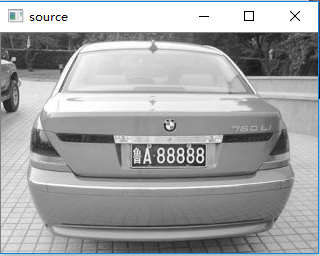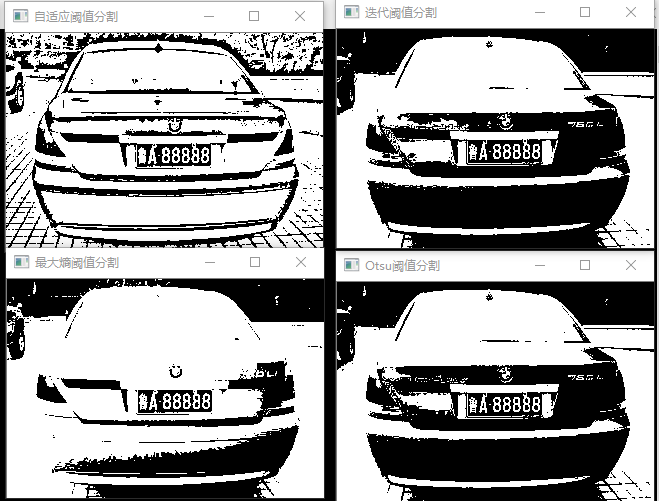展开全文opencv
• 数字图像处理—全局阈值处理 一、适合使用全局阈值处理的情况 当物体和背景的像素灰度值分布十分明显时。 二、算法实现步骤 这里使用的是迭代算法。 1.为全局阈值T选择一个初始值。（一般设为整幅图片的平均灰度值）...
数字图像处理—全局阈值处理
一、适合使用全局阈值处理的情况
当物体和背景的像素灰度值分布十分明显时。
二、算法实现步骤
这里使用的是迭代算法。
1.为全局阈值T选择一个初始值。（一般设为整幅图片的平均灰度值）
2.用T分割像素值，产生两组像素值：G1有小于等于T的像素组成；G2由大于T的像素组成。
3.分别计算G1中的平均像素灰度值T1和G2中的平均像素灰度值T2。
4.判断
|T-（T1+T2）/2|  <   △T
其中△T为预先设置的一个处置。
如果不满足条件则循环条件2-5。
5.T=（T1+T2）/2
三、代码实现
这里用的是MATLAB实现
clear
%读取图片

%计算图片的直方图分量count(i)和尺寸
[count,x]=imhist(Img);
[row,col]=size(Img);

%计算政府图片的灰度平均值T，T将图片的灰度分为类C1和类C2
sum=0;k=0;
for i=1:256
sum=sum+count(i)*(i-1);
k=k+count(i);
end
T=sum/k;
T=round(T);

%执行循环直至(T-(T1+T2)/2)小于deltaT，这里取1
flag=1;sum1=0;k1=0;sum2=0;k2=0;
while flag==1
%计算类C1的平均灰度值
for i=1:T+1
sum1=sum1+count(i)*(i-1);
k1=k1+count(i);
end
T1=sum1/k1;
T1=round(T1);

%计算类C2的平均灰度值
for i=T+2:256
sum2=sum2+count(i)*(i-1);
k2=k2+count(i);
end
T2=sum2/k2;
T2=round(T2);

if abs(T-(T1+T2)/2) <= 1
flag=0;
end

T=(T1+T2)/2;
T=round(T);
end

%将图片进行阈值为T的二分值分割
ImgT=Img;
for i=1:row
for j=1:col
if ImgT(i,j)>T
ImgT(i,j)=255;
else
ImgT(i,j)=0;
end
end
end

%显示图片
subplot(2,2,1),imshow(Img);title('原图');
subplot(2,2,[3,4]),plot(x,count);title('直方图');
subplot(2,2,2),imshow(ImgT);title('使用全局阈值分割结果图');

4.结果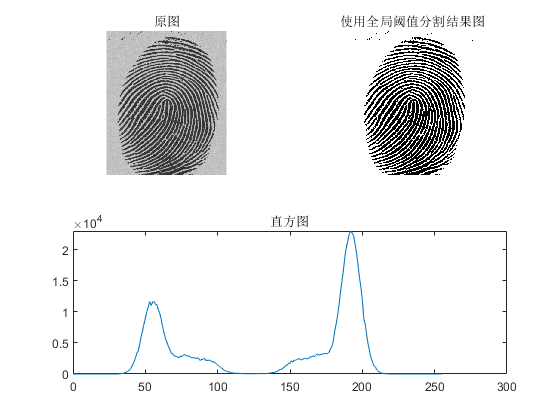展开全文数字图像处理
• 图像处理中它的意思是颜色转换的临界点，该方法只用于二值化的图像列如在自然每一种颜色都有一个值，通常由RGB（即红、绿、蓝三原色）按比例混合就会得到各种不同的颜色。阈值处理图片是对颜色进行特殊处理的...
的意思是界限，故阈值又叫临界值，是指一个效应能够产生的最低值或最高值。在图像处理中它的意思是颜色转换的临界点，该方法只用于二值化的图像中列如在自然中每一种颜色都有一个值，通常由RGB（即红、绿、蓝三原色）按比例混合就会得到各种不同的颜色。阈值处理图片是对颜色进行特殊处理的一种方法。详细说，阈值是一个转换临界点，不管你的图片是什么样的彩色，它最终都会把图片当黑白图片处理，也就是说你设定了一个阈值之后，它会以此值作标准，凡是比该值大的颜色就会转换成白色，低于该值的颜色就转换成黑色，所以最后的结果是，你得到一张黑白的图片。用阈值的作用：得到一张对比度不同的黑白图片阈值可以是最小值：某一性能特征不能低于该值。
展开全文• 图像阈值处理是实现图像分割的一种方法。 它利用图像中要提取的目标物 和 目标物的背景在灰度特性上的差异，把图像视为具有不同灰度级的两个区域（目标和背景）的组合。选取一个合适的阈值，以确定图像中的每个像素...
官网参见https://docs.opencv.org/3.4.1/d7/d4d/tutorial_py_thresholding.html
图像阈值处理是实现图像分割的一种方法。
它利用图像中要提取的目标物 和 目标物的背景在灰度特性上的差异，把图像视为具有不同灰度级的两个区域（目标和背景）的组合。选取一个合适的阈值，以确定图像中的每个像素点应该属于目标区域还是背景区域，最终产生对应的二值图像，实现图像分割。
常用阈值处理方法有：

1.简单阈值
2.自适应阈值
3.Otsu二值化

1.简单阈值
当像素值高于阈值时，它被分配一个值（例如白色），反之则被分配另外一个值（例如黑色）。opencv中实现该功能函数是 cv.threshold。
retval, dst	=	cv.threshold(	src, thresh, maxval, type[, dst]	)

src：原图像，必须是灰度图
thresh：阈值，用于对像素值进行分类
maxval：当像素值高于（或者小于）阈值时候被赋予的值
type：阈值类型，包含
cv.THRESH_BINARY
cv.THRESH_BINARY_INV
cv.THRESH_TRUNC
cv.THRESH_TOZERO
cv.THRESH_TOZERO_INV

输出retval，阈值化的图像。
例1，简单阈值设置
# -*- coding: cp936 -*-
import cv2
import numpy as np
from matplotlib import pyplot as plt

ret,thresh1 = cv2.threshold(img,127,255,cv2.THRESH_BINARY)
ret,thresh2 = cv2.threshold(img,127,255,cv2.THRESH_BINARY_INV)
ret,thresh3 = cv2.threshold(img,127,255,cv2.THRESH_TRUNC)
ret,thresh4 = cv2.threshold(img,127,255,cv2.THRESH_TOZERO)
ret,thresh5 = cv2.threshold(img,127,255,cv2.THRESH_TOZERO_INV)
titles = ['Original Image','BINARY','BINARY_INV','TRUNC','TOZERO','TOZERO_INV']
images = [img, thresh1, thresh2, thresh3, thresh4, thresh5]
for i in xrange(6):
plt.subplot(2,3,i+1),plt.imshow(images[i],'gray')
plt.title(titles[i])
plt.xticks([]),plt.yticks([])
plt.show()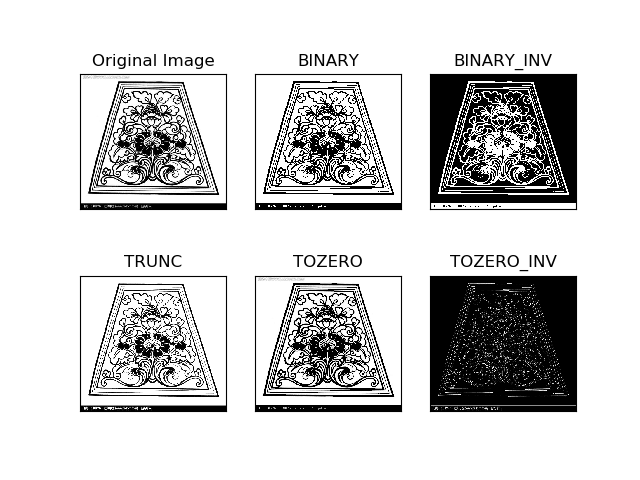不同阈值类型结果如上图。
简单阈值是把全局值作为阈值(一个图像一个阈值)，但是这不适合所有情况，特别是一个图像的不同区域亮度不同时候。此时我们就需要使用自适应阈值，该算法可以为图像的每一个小区域计算阈值。因此我们就能在同一图像的不同区域获得不同阈值，从而同一图像亮度不同的情况下获得更好的结果。
自适应阈值函数如下
dst	=	cv.adaptiveThreshold(	src, maxValue, adaptiveMethod, thresholdType, blockSize, C[, dst]	)


blockSize：相邻区域的尺寸大小
C：常数。阈值就是相邻区域平均值或者加权值减去这个常数。
例1，自适应阈值设置
# -*- coding: cp936 -*-
import cv2
import numpy as np
from matplotlib import pyplot as plt

img = cv2.medianBlur(img,5)
ret,th1 = cv2.threshold(img,127,255,cv2.THRESH_BINARY)
cv2.THRESH_BINARY,11,2)
cv2.THRESH_BINARY,11,2)
titles = ['Original Image', 'Global Thresholding (v = 127)',
images = [img, th1, th2, th3]
for i in xrange(4):
plt.subplot(2,2,i+1),plt.imshow(images[i],'gray')
plt.title(titles[i])
plt.xticks([]),plt.yticks([])
plt.show()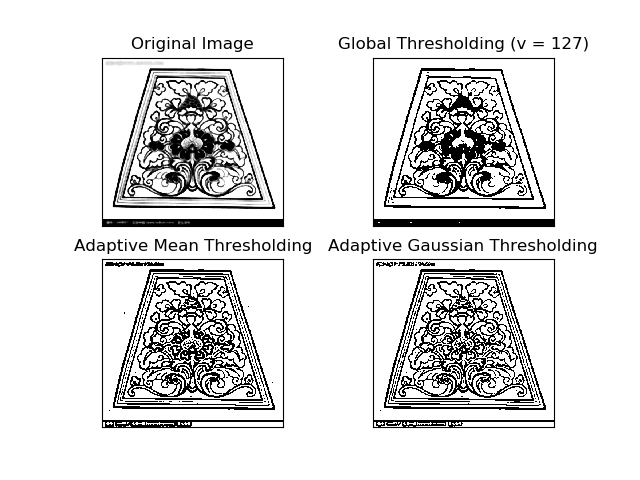block size=11，C=2，结果如上图。
medianBlur是中值滤波函数，用于图像去噪。
3.Otsu二值化
简单阈值函数cv.threshold有一个返回值retVal，我们在进行Otsu二值化时候会用到它。
简单阈值使用全局阈值时候，我们使用任意值作为阈值，那么怎么知道这个选值的好坏呢？
答案就是不停尝试。但是如果是双峰图像（直方图有2个峰值的图像）呢？
对于双峰图像，应该是取峰值中间的一个值作为阈值，这就是Otsu二值化要做的事情。
简单来说就是对双峰图像自动根据其直方图计算出阈值。但是对于非双峰图像，二值化结果则不够精确。
Otsu二值化在opencv中还是使用cv.threshold函数，阈值类型为cv.THRESH_OTSU。
此时需要把阈值thresh设置为0。算法会找到最优阈值，并在返回在retVal中。如果不适用Otsu二值化，那么retVal=threshold。
例，Otsu二值化
import cv2 as cv
import numpy as np
from matplotlib import pyplot as plt

# global thresholding
ret1,th1 = cv.threshold(img,127,255,cv.THRESH_BINARY)

# Otsu's thresholding
ret2,th2 = cv.threshold(img,0,255,cv.THRESH_BINARY+cv.THRESH_OTSU)

# Otsu's thresholding after Gaussian filtering
blur = cv.GaussianBlur(img,(5,5),0)
ret3,th3 = cv.threshold(blur,0,255,cv.THRESH_BINARY+cv.THRESH_OTSU)
# plot all the images and their histograms
images = [img, 0, th1,
img, 0, th2,
blur, 0, th3]
titles = ['Original Noisy Image','Histogram','Global Thresholding (v=127)',
'Original Noisy Image','Histogram',"Otsu's Thresholding",
'Gaussian filtered Image','Histogram',"Otsu's Thresholding"]
for i in xrange(3):
plt.subplot(3,3,i*3+1),plt.imshow(images[i*3],'gray')
plt.title(titles[i*3]), plt.xticks([]), plt.yticks([])
plt.subplot(3,3,i*3+2),plt.hist(images[i*3].ravel(),256)
plt.title(titles[i*3+1]), plt.xticks([]), plt.yticks([])
plt.subplot(3,3,i*3+3),plt.imshow(images[i*3+2],'gray')
plt.title(titles[i*3+2]), plt.xticks([]), plt.yticks([])
plt.show()

该代码直接来自官网。
它输入的图像是一个带有噪声的图像。其中使用了3种方法获得阈值化图像。
第1种方法，简单阈值，设127 为全局阈值。
第2种方法，直接使用Otsu二值化。threshold=0
第3种方法，首先使用一个5x5 的高斯核过滤噪音，然后再使用Otsu 二值化。
看看噪音过滤对结果的影响有多大吧。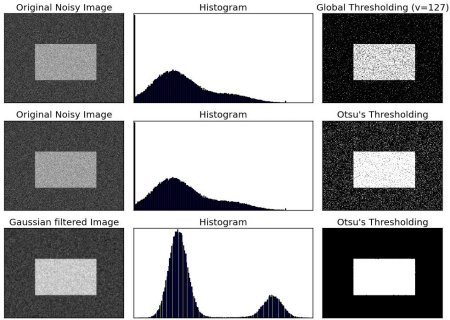以上是运行结果，我直接从官网复制了。


展开全文• 全局阈值就是在整个图像中将灰度阈值设置成一个常数，全局阈值适合如下场景：图像背景的灰度值在整个图像中可合理的看作恒定，而且所有物体和背景都具有几乎相同的对比度，反应在直方图上就是 具有明显的双峰。...OpenCV
• 该系列文章是讲解Python OpenCV图像处理知识，前期主要讲解图像入门、OpenCV基础用法，中期讲解图像处理的各种算法，包括图像锐化算子、图像增强技术、图像分割等，后期结合深度学习研究图像识别、图像分类应用。...Python 图像处理 OpenCV 阈值化处理 threshold
• 我们将图像分块最简单的方法就是设定一个阈值图像进行二值化处理，那么这个阈值我们应该如何选择呢 对于图像的直方图存在明显边界的图像，我们可以很容易找到这个阈值，但是如果图像直方图分界不明显，那么这个...数字图像处理 阈值 自适应阈值 OTSU算法
• 基本概念 图像阈值分割是图像处理中最基本也是最常用的方法之一，主要用于将图像的像素点划分为两个或者多个类别，从而得到便于处理的目标对象。 ...阈值分割
• ## 图像处理基本算法 动态阈值分割

万次阅读 多人点赞 2012-02-11 16:18:32
图像处理时，受外界光线的干扰一般比较大，假如在阈值分割时采用固 定阈值，那么在环境改变时分割效果受影响极大，那么为了避免此影响就 必须采用动态阈值，自动求出合适的阈值进行分割。 本文的介绍几...算法 图像处理 image 优化图像处理
•  图像处理中很重要的概念就是图像分割，在很多应用都需要图像分割的处理，例如产品检测，目标识别，匹配等。图像分割的概念，我之前在其他博客描述过，分割：就是在一幅图像，提取出感兴趣区域的过程。主要有四...MATLAB
• 在前面的部分使用是全局阈值，整幅图像采用同一个数作为阈值。当时这种方法并不适应与所有情况，尤其是当同一幅图像上的不同部分的具有不同亮度时。这种情况下需要采用自适应阈值。此时的阈值是根据图像上的每一个小...Python opencv 图像处理
• 目录 1 什么是阈值化-threshold() ...图像的二值化或阈值化 (Binarization)旨在提取图像中的目标物体，将背景以及噪声区分开来。通常会设定一个阈值，通过阈值图像的像素划分为两类：大于阈值的...数字图像处理 Python
• 基本的全局阈值处理图像的前景和背景相关的只方图之间存在一个相当清晰的波谷时，这个算法效果很好。这部分算法比较简单，由于时间关系，并没有写相关代码算法步骤：一、为全局阈值T选择一个初始的估计值（一般选...
• 注意：应用灰度直方图双峰法来分割图像，也需要一定的图像先验知识，因为同一个直方图可以对应若干个不同的图像，直方图只表明图像中各个灰度级上有多少个象素，并不描述这些象素的任何位置信息。 该方法不...数字图像处理 图像分割
• %迭代式阈值分割 otsu阈值分割 二值化 close all;%关闭所有窗口 clear;%清除变量的状态数据 clc;%清除命令行 I=imread('rice.png'); subplot(2,2,1); imshow(I); title('1 rice的原图'); %迭代式阈值分割 zmax=...matlab 图像处理
• ## Matlab实现图像阈值分割

万次阅读 多人点赞 2019-08-03 11:32:54
使用matlab实现阈值分割，实现两种方法，一是人工选择阈值进行分割，而是自动选择阈值进行分割。操作步骤 1、 打开Matlab内容自带的coins.png图像。 2、 观察它的直方图。 3、 人工选定一个阈值，并进行分割。 4...matlab 图像处理 阈值分割算法
• 图像阈值处理一般使得图像的像素值更单一、图像更简单。阈值可以分为全局性质的阈值，也可以分为局部性质的阈值，可以是单阈值的也可以是多阈值的。当然阈值越多是越复杂的。下面将介绍opencv下的三种阈值方法。...opencv python
• 参照下图实现对指纹图像的基本全局阈值分割。 二.实验分析 计算基本全局阈值算法思想如下: ① 选择一个T的初始估计值②用T分割图像，生成两组像素：G1由所有灰度值大 于T的像素组成，而G2由所有灰度值小于...图像处理 算法
...